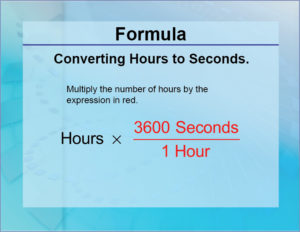# Java: Convert Hours To Seconds & Minutes | Vice Versa

Java program to convert hours to minutes & seconds vice versa. Its a simple code to convert hours to seconds, this java program has been written in 4 ways for easy understanding. If you have any queries just do leave a comment here.

In this tutorial, we learn how to use the Java program to effectively to calculate and convert the number of hours into seconds and it will involve us using the scanner class and a few tweaks here and there. So let’s quickly delve into the problem solving with Java.## Java to convert Hours into Seconds

Converting hours to seconds is quite simple. All we require is an input denoting the hours.

We are well aware that, one minute is 60 seconds and one hour is 60 minutes which will make, one hour to 3600 seconds (60*60). This same logic is used to convert hours to seconds.

For this, we need to read our input hours at runtime. This can be done by using Scanner class which is well known for reading any primitive datatype at runtime from the console screen.

So, we’ll first create an object of this Scanner class and then invoke the required method to read our input. Since our input is hours, which is generally an integer only, we will be making use of the nextInt() method of the class by writing the following statements:

Now that we have our input in hand, since 1 hour == 60*60 seconds or 3600 seconds

x hours == x*60*60 seconds by cross multiplication.

Therefore our output seconds will be calculated as:

sec=hrs*60*60;

Output:

Using Method

In this method, we’ll be making use of another method which will consist of the main logic for converting hours to seconds i.e.,

sec=h*60*60;

We are making use of another separate method so that, we can make the main logic of the code reusable.

So, we first read the input hours using Scanner class and then create an object (sc) of this method.

Since it is not within main method, object is required to invoke it.

Then with this created object (res) of the class (hoursToSceonds) we invoke the seconds() method by passing hours as parameter which performs calculation and stores resultant is a variable (sec).

This variable (sec) is then called in the main method using the same object to be printed on console screen using println() method.

Output:

Using Static Method

Similar to the above method, even here we write the code is a separate method. The only difference is that, we make use of static method here instead.

The advantage of static method is that, we do not have the need to create an object for static method even though,

the static method is outside main method because, it belongs to the class instead of the instance of the class.

So now, the static method (hrsToSec) contains the logic while the main method takes care of input output operations and call this static method (hrsToSec) by passing input hours as argument.

### Java Code Hours To Minutes – Using Command Line Arguments

In all the above methods, we have seen the use of Scanner class to read input at runtime.

Similarly, we can also make use of command line arguments to read input at runtime.

Here, while we write the run command itself we send our input as argument with a space in between.

This argument is generally of type string. So, we first convert it to desired type (integer) by parsing. This is then stored in a variable followed by which, the same logic as above is used.

Since there is only one argument (arg), we parse this as above which is our required input hours.

x

## Java Program to Add Two Matrices – 4 Ways | Programs

Java program to add two matrices – The following Java Code will let you know ...